Trial ends in

A subscription to JoVE is required to view this content.
You will only be able to see the first 20 seconds.

Force and Acceleration
Energy and Work
Enthalpy

# Force and Acceleration

### Overview

Source: Nicholas Timmons, Asantha Cooray, PhD, Department of Physics & Astronomy, School of Physical Sciences, University of California, Irvine, CA

The goal of this experiment is to understand the components of force and their relation to motion through the use of Newton's second law by measuring the acceleration of a glider being acted upon by a force.

Nearly every aspect of motion in everyday life can be described using Isaac Newton's three laws of motion. They describe how objects in motion will tend to stay in motion (the first law), objects will accelerate when acted upon by a net force (the second law), and every force exerted by an object will have an equal and opposite force exerted back onto that object (the third law). Almost all of high school and undergraduate mechanics is based on these simple concepts.

### Principles

One of the most famous equations in all of physics is Newton's second law: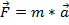. (Equation 1)

It simply states that the force on an object is equal to the mass of the object times its acceleration.

In the experiment to follow, a glider will be connected to a falling weight by a pulley. Because friction caused by the glider sliding along a track would result in an extra force that is difficult to measure, the glider will be on an air track to reduce friction. The air track creates a cushion of air between the glider and track, reducing any friction to approximately zero. The force of the weight will accelerate the glider according to Equation 1.

The force on the weight will be due to gravity and the tension in the string connecting the falling weight to the glider. The tension will oppose the direction of the falling weight and will have the opposite sign as the force of gravity in the equation. Thus, Equation 1 becomes, where T is the tension and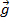is the acceleration due to gravity (~9.8 m/s2). While the acceleration due to gravity will remain the same, the force can be increased by adding mass.

As the weight falls, it creates tension in the string connecting the weight to the glider. The pulley changes the direction of the tension force from vertical to horizontal. With nothing else connected, the tension in the string is equal to the force of the falling weight, which applies the same magnitude of force to the glider. Therefore, the force on the glider will be equal to the tension force T;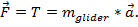Because the weight and the glider are connected, their acceleration will be the same for both objects. To calculate the acceleration on the glider due to the pull of the weight, the forces are equated.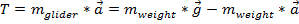, which can be solved for :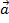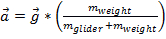. (Equation 2)

To measure the acceleration, a photogate timer is placed 20 cm from the initial position of the glider. The acceleration can be calculated from the measured final velocity and distance traveled using the following equation: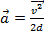, (Equation 3)

where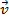is the final velocity andis the distance traveled. The flag at the top of the glider will pass through the photogate, which will record the amount of time the glider takes to pass through the gate. The flag is 10 cm long, so the velocity of the glider is equal to the length of the flag divided by the time.

### Procedure

1. Initial setup.

1. The air track will have a pulley connected to one end. Tie the string to one end of the glider and run it through the pulley, where it will be connected to the hanging weight.
2. Place the glider at the 190-cm mark on the air track. Place the photogate timer at the 100-cm mark. The glider itself has a mass of 200 g. Hold onto the glider so that it does not move and add weights to the hanging end so that the total mass of the weight is equal to 10 g.
3. Once the weights are in place, release the glider from rest and record the velocity of the glider. Perform 5 runs and take the average value.
4. Calculate the theoretical value for acceleration using Equation 2 and the experimental value from Equation 3. For example, if the glider has mass of 200 g and the hanging weights have a mass 10 g, then the theoretical acceleration, from Equation 2, is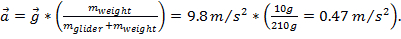If the measured velocity is 0.95 m/s, then, using Equation 3, the experimental value for acceleration is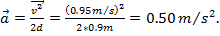2. Increasing the mass of the glider.

1. Add four of the weights to the glider, which will double its mass.
2. Release the system from rest and record the velocity of the glider. Perform 5 runs and take the average value. Calculate the theoretical value for acceleration, from Equation 2, and the experimental value, from Equation 3.

3. Increasing the force on the glider.

1. Add more mass to the hanging weight so that it has a total mass of 20 g.
2. Release the system from rest and record the velocity of the glider. Perform 5 runs and take the average value.
3. Calculate the theoretical value for acceleration, from Equation 2, and the experimental value, from Equation 3.
4. Add more mass to the hanging weight so that it has a total mass of 50 g.
5. Release the system from rest and record the velocity of the glider. Perform 5 runs and take the average value.
6. Calculate the theoretical value for acceleration, from Equation 2, and the experimental value, from Equation 3.

Newton's second law describes the relationship between force and acceleration and this relationship is one of the most fundamental concepts that apply to many areas of physics and engineering.

F equals ma is the mathematical expression of Newton's second law. This illustrates that greater force is required to move an object of a larger mass. It also demonstrates that for a given force acceleration is inversely proportional to mass. That is, with the same applied force smaller masses accelerate more than larger masses

Here we will demonstrate an experiment that validates Newton's second law by applying forces of different magnitudes to a glider on a nearly frictionless air track

Before going into the details of how to run the experiment, let's study the concepts and laws that contribute to the data analysis and interpretation.

The set-up consists of an air track, a glider, a photogate timer at a known distance d from the starting point, a pulley, and a string running from the glider over the pulley.

If one attaches a weight to the other end of the string and releases it, the weight will apply a force on the glider causing it to accelerate. This force is given by Newton's second law. At the same time, the force on the weight will be due to gravitational acceleration minus the tension force in the string connecting the falling weight to the glider. This tension force is the mass of the weight times the acceleration of the glider.

By equating the force on the glider with the force on the weight, one can derive the formula to theoretically calculate glider's acceleration.

The experimental way to calculate the glider's acceleration is with the help of the photogate timer. This gives us the time taken by the glider to travel distance d from the starting point. Using this information, one can calculate the glider's speed and then, with the help this kinematics formula, one can calculate the magnitude of experimental acceleration.

Now that we understand the principles, let's see how to actually conduct this experiment in a physics lab

As mentioned before, this experiment uses a glider connected by a line passing over a pulley to a weight. The glider slides along an air track, which creates a cushion of air to reduce friction to negligible levels.

As the weight falls, the pulley redirects the tension in the line to pull the glider, which has a 10 cm long flag on top. A photogate at a known distance from the starting point records the amount of time it takes for the flag to pass through it

The glider's final velocity is the length of the flag divided by the time to pass through the photogate. With the glider's final velocity and the distance traveled, it is possible to calculate acceleration.

Set up the experiment by placing the photogate timer at the 100 cm mark on the air track and the glider at the 190 cm mark. The glider has a mass of 200 grams. Hold the glider so it does not move and add weights to the end of the string so the total hanging mass is also 10 grams

Once the weights are in place, release the glider, record its velocity for five runs and calculate the average. Use the mass of the glider and the hanging weight to calculate the experimental and theoretical accelerations then record the results.

Now add four more weights to the glider, doubling its mass to 400 grams. Place the glider at the 190 cm mark to repeat the experiment. Release the glider and record its velocity for five runs. Again, calculate and record the average velocity and the experimental and theoretical accelerations.

For the last set of tests, remove the weights from the glider so it has its original mass of 200 grams. Then, add weights to the hanging mass until it has a new mass of 20 grams. Repeat the experiment for another five runs.

Finally, add more weights to the hanging mass until it is 50 grams and repeat the experiment for five more runs.

Recall, the theoretical acceleration of the glider is equal to the acceleration due to gravity g multiplied by the ratio of the mass of the falling weight and the mass of the weight and glider together. As the theoretical values on this table show, acceleration decreases as the mass of the glider increases.

Conversely, acceleration increases as the mass of the falling weight increases, due to the greater force. Note that the accelerations predicted by this equation can have a maximum value of g, which is 9.8 meters per second squared.

Next, let's see how to calculate the experimental acceleration. For example, the first test used a 200-gram glider and a 10-gram weight. The average speed after traveling 100 centimeters was 0.93 meters per second. Using the kinematics equation discussed before, the experimental acceleration comes out to be 0.43 meters per second squared. This same calculation, applied to the other tests, produces the results shown on this table.

The differences between experimental and theoretical accelerations may have several causes, including limitations in measurement accuracy, the very small but not completely negligible friction on the air track, and the air pocket beneath the glider, which may add to or subtract from the force of tension along the string.

Forces are present in almost all phenomena in the universe. Brought down to Earth, forces affect all aspects of daily life.

Striking the head may cause trauma and impair cognitive functions. A study of sports related concussions used special hockey helmets fitted with three-axis accelerometers to measure acceleration during impact.

Data were sent by telemetry to laptop computers, which recorded the measurements for later analysis. Knowing the accelerations and the mass of the head, it was possible to use Newton's second law, F=ma, to calculate the impact forces on the brain.

Civil engineers building footbridges are interested in studying the effect of force induced by foot load on these structures. In this study, the researchers placed sensors on a footbridge that measured vibrations induced by the pedestrians. The structural response was then measured in terms of vertical acceleration, which is an important parameter in studying the stability of these structures

You've just watched JoVE's introduction to force and acceleration. You should now understand the principles and protocol behind the lab experiment that validates Newton's second law of motion. As always, thanks for watching!

### Results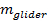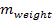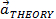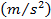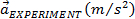% Difference 200 10 0.93 0.47 0.43 9 400 10 0.66 0.24 0.22 9 200 20 1.28 0.89 0.82 9 200 50 1.96 1.69 1.92 145

The results of this experiment confirm the predictions made by Equations 2 and 3. With the increased mass of the glider in step 2, the acceleration was smaller because a larger force would be needed to accelerate the glider to the same velocity as in step 1. In step 3, the increased mass of the hanging weight did indeed increase the force on the glider and thus the acceleration. The acceleration increased with the increased mass, as predicted.

Friction was nearly zero thanks to the air cushion between the glider and the track. The pocket of air is not perfect, however, and the air from the track might push the glider in a specific direction. This can be tested by allowing the glider to sit on the air track, with no force applied to it. If the glider moves in either direction, there might be some force on the glider from the track.

### Applications and Summary

Newton's second law is fundamentally linked to the motion people experience every day. Without any force, an object will not accelerate and will remain at rest or will continue to move at a constant rate. Therefore, if someone wants to move something, such as when hitting a baseball a certain distance, sufficient force must be applied. The force can be calculated with an equation as simple as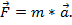Just as it takes a certain force to accelerate an object, it takes the same amount of force to bring the velocity of an object down to zero. By looking at, it is clear that an abject with a lot of mass is much harder to stop than an object with a smaller mass. It is easier to stop a bike than a train! The faster something is going, the more acceleration is required to bring it to a stop, so it takes much more force to stop a bullet than a basketball.

Newton's second law becomes a bit more complicated when the components of force change with time. For an object that is experiencing some kind of drag force, such as air resistance, its acceleration can change with time. A rocket is an example of an object that has a mass that changes with time. As the rocket burns fuel, its mass gets smaller, and it actually requires less force to accelerate as time passes.

In this experiment, the components of force were examined. Newton's second law states that force is equal to the mass of an object multiplied by the acceleration. By adjusting the mass of the glider, the acceleration of the glider was reduced. With increased force on the glider, the acceleration was increased, confirming Newton's second law. The results of this experiment should be accurate, as long as there are no other forces acting on the glider. This is why friction was reduced in this experiment using an air track.X